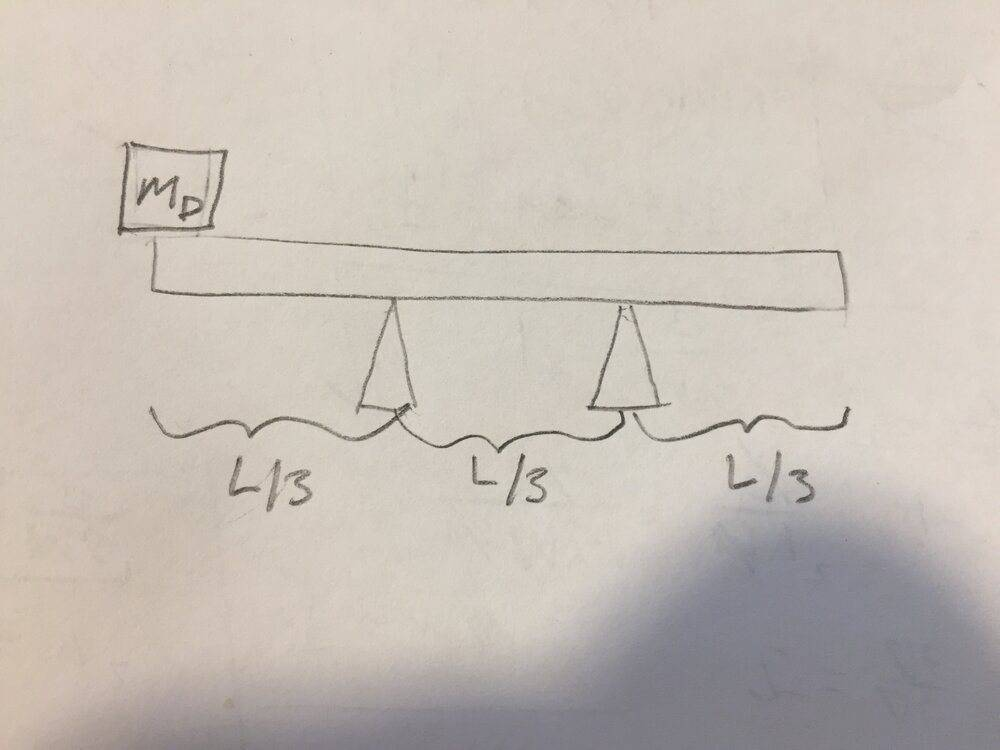# Static equilibrium: mass on beam

• jkfjbw
In summary, the linear second law for static equilibrium states that the sum of the forces in the y direction is zero. However, when solving for the force in the y direction using Newton's linear second law, you must also take into account the weight of the bar.

#### jkfjbw

Homework Statement
A horizontal bar of length ##L## and mass ##m_B## is on two fulcrums, one ##L/3## from the left side of the bar, and the other ##L/3## from the right side of the bar. There is also a mass of mass ##m_D## on the left end of the bar. Find the forces that the fulcrums exert on the bar.
Relevant Equations
$$\sum_i \vec{F}_i = \vec{0}$$
$$\sum_i \tau_{z,i} = 0$$
A diagram of the physical situation is below:Choosing the positive ##y## direction to be upwards and the positive direction of rotation to be counterclockwise, Newton's linear second law gives:
$$-m_D g + F_L + F_R = 0$$
where ##F_L## is the magnitude of the force exerted on the bar by the left fulcrum, and ##F_R## is the magnitude of the force exerted on the bar by the right fulcrum. Taking the point of rotation to be around the left fulcrum, Newton's rotational second law gives:
$$(m_D g)\Big(\frac{L}{3}\Big) + F_R\Big(\frac{L}{3}\Big) - (m_B g)\Big(\frac{L}{6}\Big) = 0\\ m_D g + F_R - \frac{1}{2} m_B g = 0\\ F_R = g\Big(\frac{1}{2}m_B - m_D\Big)$$
which is the correct answer for ##F_R##. The problem comes when I try to find ##F_L## by using Newton's linear second law to eliminate ##F_R## and solve for ##F_L##. Doing so gives:
$$m_D g + m_D g - F_L - \frac{1}{2}m_B g = 0\\ F_L = 2m_D g - \frac{1}{2}m_B g\\ F_L = g\Big(2m_B - \frac{1}{2}m_B\Big)$$
which is the wrong answer for ##F_L##.

Likewise, I can also take the point of rotation to be around the right fulcrum:
$$(m_D g)\Big(\frac{2}{3}L\Big) - F_L\Big(\frac{L}{3}\Big) + (m_B g)\Big(\frac{L}{6}\Big) = 0\\ 2m_D g - F_L + \frac{1}{2}m_B g = 0\\ F_L = g\Big(2m_D + \frac{1}{2}m_B\Big)$$
which is the correct answer for ##F_L##. However, if I use Newton's linear second law to eliminate ##F_L## and solve for ##F_R##, I get the wrong answer:
$$2m_D g - m_D g + F_R + \frac{1}{2}m_B g = 0\\ m_D g + F_R + \frac{1}{2}m_B g = 0\\ F_R = -g\Big(m_D + \frac{1}{2}m_B\Big)$$

Why do I get the wrong answer for ##F_L## and ##F_R## when I substitute Newton's linear second law into the known-correct answer for ##F_R## and ##F_L##?

Last edited:
Your static equilibrium equation for summed forces in the y direction is wrong. You are leaving out some forces. Watch your subscripts also for mb vs. md. And check your algebra.

•Lnewqban
Ah yes, I forgot the weight of the bar in Newton's linear second law. Thank you.